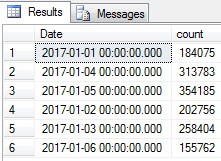## MSSQL Count by Date (when column is DateTime)

In Microsoft SQL, how can we group by a date, when the column we want to group by is a date time?
For example, you want the number of rows created for each date, and you have a column in the database called Date_Added (or in my example below, TrcDateTime).

Code Sample 1:

``````<pre>
select DATEADD(dd, 0, DATEDIFF(dd, 0, trcDateTime)) as Date,
count(*) as count
from trace
GROUP BY DATEADD(dd, 0, DATEDIFF(dd, 0, trcDateTime))
</pre>``````

Output 1:The above gives the correct counts, but gives a rather  ugly Date, because it shows the time as 00:00:00.00.0000. To remove that, we can convert the date to a string.

Code Sample 1:

``````<pre>
select LEFT(CONVERT(VARCHAR, DATEADD(dd, 0, DATEDIFF(dd, 0, trcDateTime)), 120), 10)  as Date,
count(*) as count
from trace
GROUP BY DATEADD(dd, 0, DATEDIFF(dd, 0, trcDateTime))
</pre>``````

This is how you can convert a DateTime to a String:

LEFT(CONVERT(VARCHAR, trcDateTime, 120), 10)

I took the entire expression from the first query: DATEADD(dd, 0, DATEDIFF(dd, 0, trcDateTime))
and substituted it where the italic trcDateTime was in the expression above.